Square Roots 6
Set 6 (10 Questions)
Students use exponents, powers, and roots, and use exponents in working with fractions
From Mr. Anker Tests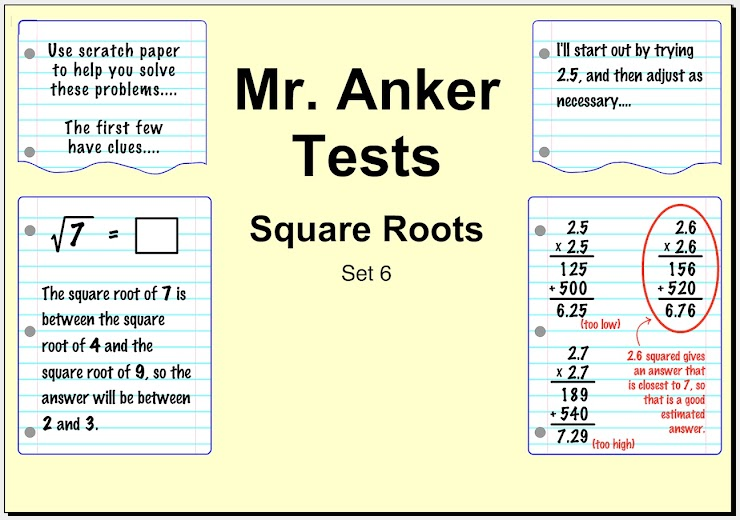Square Roots Help Slide 1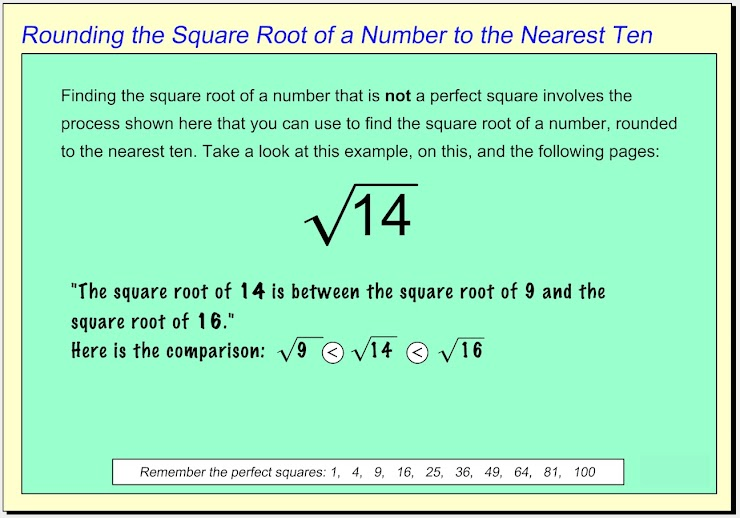Square Roots Help Slide 2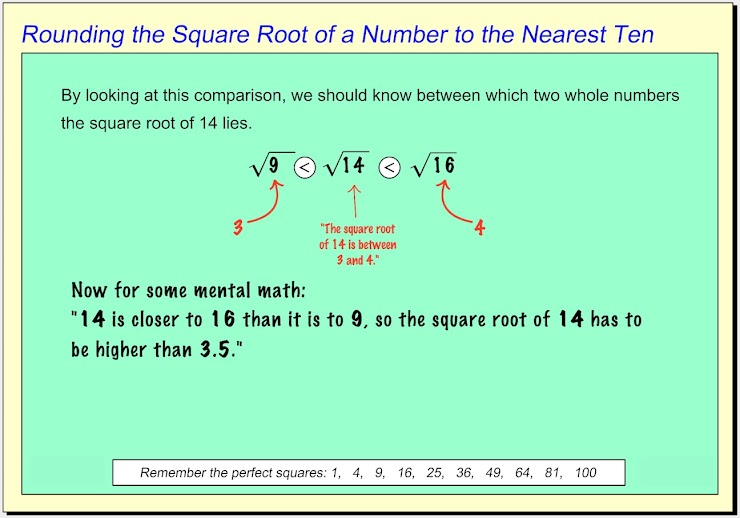Square Roots Help Slide 3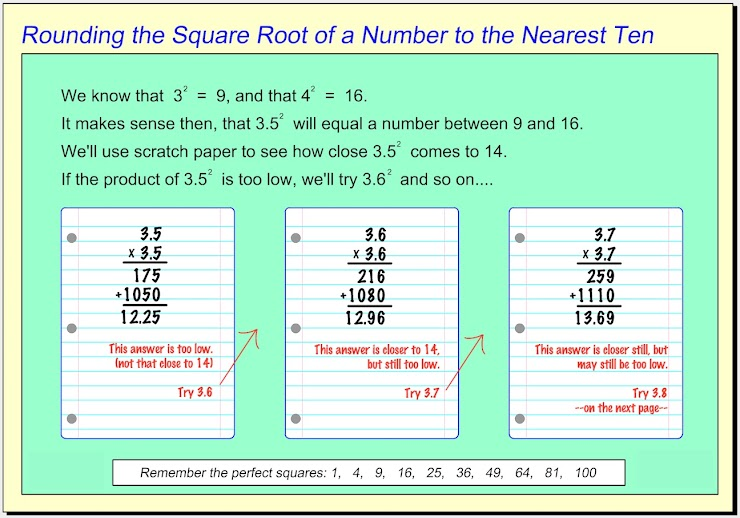Square Roots Help Slide 4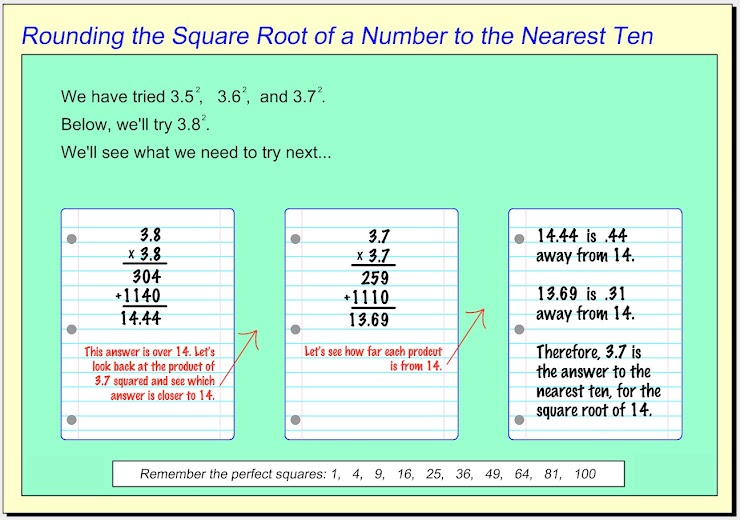Square Roots Help Slide 5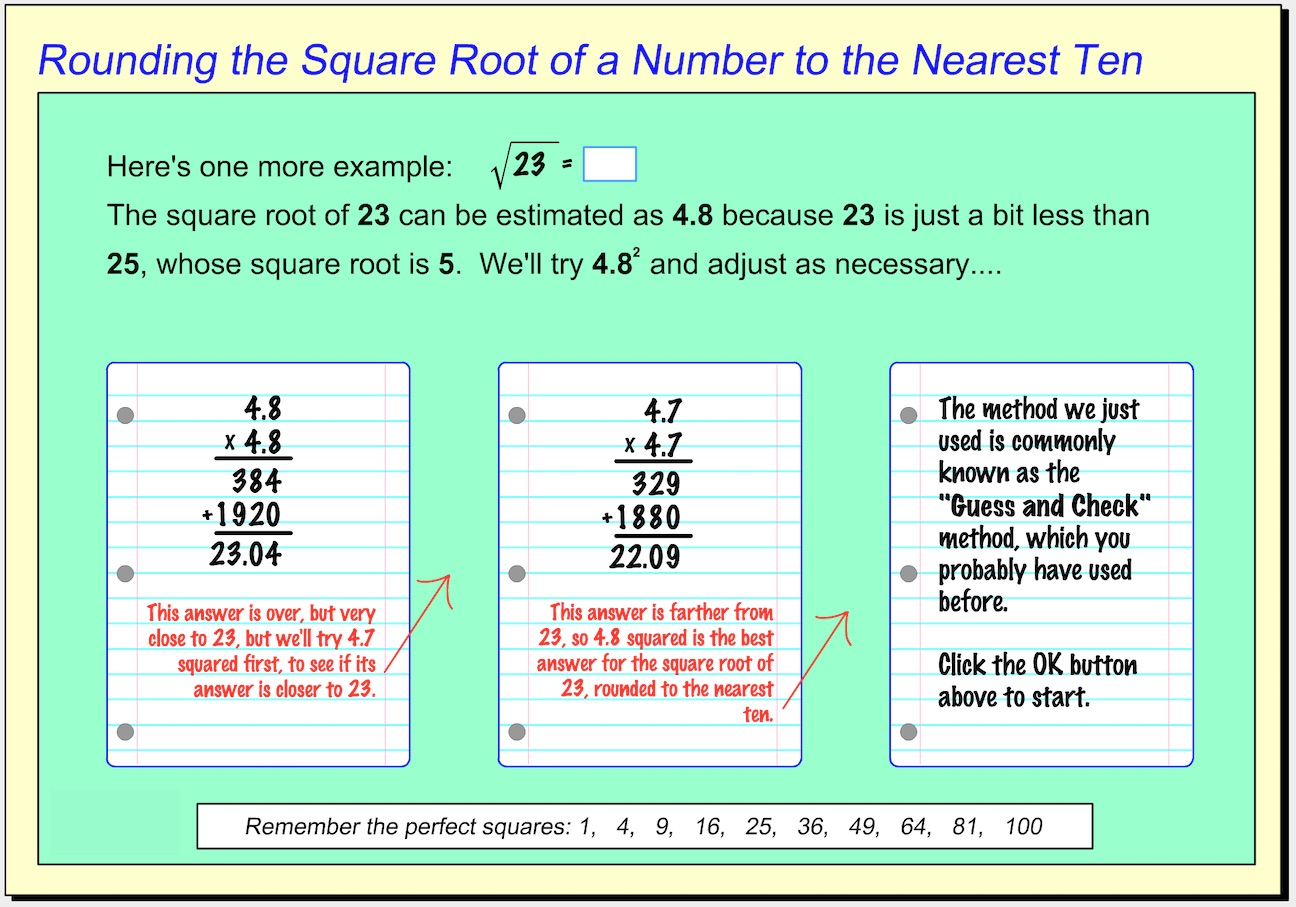1.  Calculate the square root of  8  to the nearest 10th (tenth).  Which answer choice is the closest? *
1 point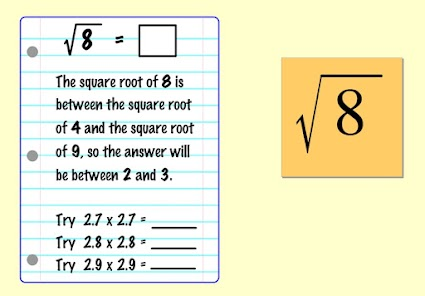2.  Calculate the square root of  13  to the nearest 10th (tenth).  Which answer choice is the closest? *
1 point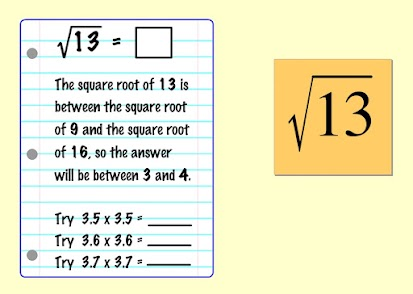3. Calculate the square root of  17  to the nearest 10th (tenth).  Which answer choice is the closest? *
1 point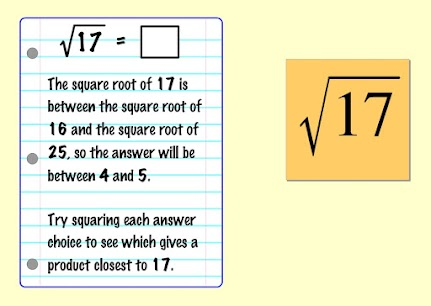4. Calculate the square root of  19  to the nearest 10th (tenth).  Do the work on scratch paper. Then type your answer on the line below. *
1 point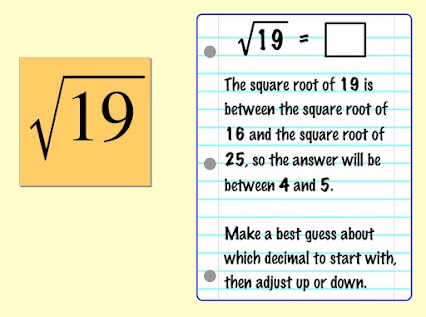5.  Calculate the square root of  23  to the nearest 10th (tenth).  Do the work on scratch paper. Then type your answer on the line below. *
1 point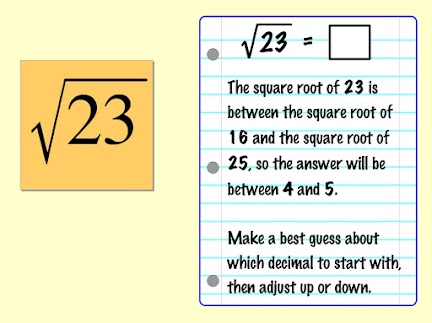6.  Calculate the square root of  28  to the nearest 10th (tenth).  Do the work on scratch paper. Then type your answer on the line below. *
1 point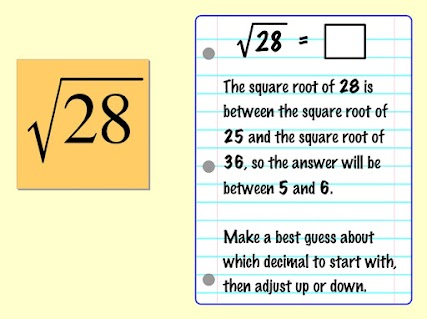7.  Calculate the square root of  35  to the nearest 10th (tenth).  Do the work on scratch paper. Then type your answer on the line below. *
1 point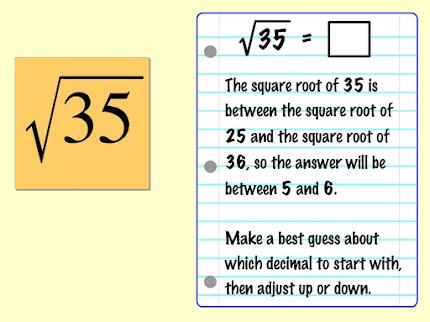8.  Calculate the square root of  44  to the nearest 10th (tenth).  Do the work on scratch paper. Then type your answer on the line below. *
1 point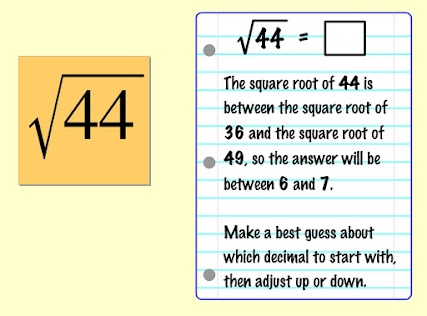9.  Calculate the square root of  67  to the nearest 10th (tenth).  Do the work on scratch paper. Then type your answer on the line below. *
1 point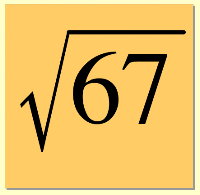10.  Calculate the square root of 82 to the nearest 10th (tenth). Do the work on scratch paper. Then type your answer on the line below. *
1 point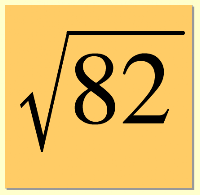Submit
Clear form